Mathmatics revision guide Gcses to additional maths^^ i am yr 11 ad maths grp plz ask me questions take it in mind im nt a genius tho =oMathmatics revision guide Gcses to additional maths^^ i am yr 11 ad maths grp plz ask me questions take it in mind im nt a genius tho =o

Algebra (yr6-7)
Algebra is one of the easiest topics to come about, it varies through questions such as
find x:
2x=2
x means a number which is multiplied by 2 = 2 therefore we divide the 2x by 2 which equals...
1!
wasn't that easy =)
lets try HARDER one
2x-5 = 10
theres a minus 5 this time =o what do we do?
you simply carry -5 against the equal sign which makes it a plus.
2x = 15
now its much clearer^^
x = 15
2
x = 7.5
that is pretty much all you need for algebra at this stage... if you feel this is waay too easy check out some higher level stuff^^

Last edited by 016016 on Wed Jan 18, 2012 4:56 am; edited 1 time in totalwavey
Heavyweight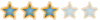Posts : 108
Join date : 2012-01-17
Age : 23
Location : ...secretmathmatic revision algebra yr 8-10

at yr 8-10, it starts to get more difficult..
first thing you do rearrange this to get the subject to x.
3x+29 = 6x - 1
all we do is cross the -1 to the + 29...
remember when there is an equal sign, you do the opposite of the sign.
so u get
3x + 30 = 6x.... take 3x over to 6x...
30 = 3x
3x = 30 change it around to get 3x at left hand side
x=10 done!

Rearranging subject seems harder than it truly is^^
change the subject to x
t = abc
x
here, you simply carry the x to the t... remember that x is dividing the abc so it becomes a times by t.
tx = abc
now simply just take t over abc this time because t is timesing the x, when carried over becomes divide.
x = abc
t
easy peazy^^
now for some harder work...
tx = go-x
r
we move the r to tx like the previous question.
rtx = go-x
now move the x to the other side
rtx + x = go
this is the clever part.
factorise the rtx + x so that only x is on its own
x(rt+1) = go
now we can just get the brackets to the =go!
x = go
rt+1
and that is all there is to it^^wavey
HeavyweightPosts : 108
Join date : 2012-01-17
Age : 23
Location : ...secreteasy version of solving fractions... division

I always found it hard to work out divisions in a fraction and my teacher was like wtf.. its so ez... she showed me how to do it^^ and ill do the same to show how easy it can be~
lets look t this example.
2 / 4
3 5
now the trick is...
... swap the 2nd numbers around, the ned and the donkey... (numerator and the denominator)
the add a cross...
2 X 5 ( remember if you are multiplying fractions, just multiply top nb and bottom number
3 4 as it is)
so you get
10/12
which is the answer =)
clever isnt it? xD
2/3 = 0.66.
4/5 = 0.8
= 10/12 =)
easy~!wavey
HeavyweightPosts : 108
Join date : 2012-01-17
Age : 23
Location : ...secretAdditional maths~ differentiation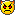differentiation is just about additional maths gcse... it appears frequently on Alevel maths.
you first of all get a question like
y = 12x(1) + 3x(2) -2x(3) () means squared or cubed.
first of all bring down the squared sign to the front number like so by taking down a sqr each time...
dy = 12 + 6x - 6x(2)
dx
now swap it round so it becomes
dy= 6x(2) - 6x -12
dx
from this... do what wecan do, cancel out the ugly numbers to smaller ones =)
dy = (x(2) - x - 2)
dx
which can be factorised to
x =2 x = -1
this work is usually to do with graphs... there are 4 more parts to this... + integration which is the opposite~ keep tuned^^wavey
HeavyweightPosts : 108
Join date : 2012-01-17
Age : 23
Location : ...secreteasy indicies

this topic is very easy but it comes and goes through the year.
basics in which you need to know is when ur dividing an indicies, you take away and when ur multiplying u add =)
6(20) / 6(15) = 6(5)
6(30) x 6(10) = 6(40)
there not so hard =')wavey
HeavyweightPosts : 108
Join date : 2012-01-17
Age : 23
Location : ...secretrules of trigonometry

there are 3 parts to a triangle a b c or adjacent opposite and hypotenuse.
basic trig question is adjacent and opposite sides are 3 and 4, find the hypotenuse x.
this is easy and you need to remember it to get easy marks.
when youre asked to find hypotenuse of this question u must remember its 3 4 5 =)
this is the most simple form you will probably find.
but how do you work it out?
well hypotenuse means the longest side and there is a mathematical formula to find this out.
adjacent squared + opposite squared will always equal to the hypotenuse squared.
so in this case 3(2) + 4(2) = 5(2).
9 + 16 = 25.
now why is 3 4 5 simple?
well 3 4 5 is simple because its multipliers works the same way.
for example, 6 8 10 works
36 + 64 = 100
and im sure 9 12 15 works as well.
look for these shortcuts to extend the time to look at other hard questions.wavey
HeavyweightPosts : 108
Join date : 2012-01-17
Age : 23
Location : ...secretSolving inequalities this is acutally A level.. lol! its a topic cant miss a mark on folks!

this is in algebraic questions sometimes... its actually as easy as normal expansion and factorization...just watch =)
20-x < 2(6x+5)
20 - x < 12x+10
10 < 13x
10 < x
13
but sometimes the examiner wants you to swap the croc clips around...
x > 10
13
arrow means V
bigger number > smaller number
smaller number < bigger number
bigger than 0 > 0 > smaller than 0
arrows with a dash underneathe just means greater or smaller than or equal to.
just remember it like crocodile eats bigger prey... thats all i can say on that topic.wavey
HeavyweightPosts : 108
Join date : 2012-01-17
Age : 23
Location : ...secretCompleting the square all the way up to A level.

here we have a quadratic expression.
x2+20x
we want them in brackets =3
but how? we can't factorize it...
or can we?
what we do here is split the 2nd number... the +20 in to 2 and put it in brackets.
so
(x+10) (x+10) now this gives us x2 + 20x + 100
now all we have to do is make it the same as the first number... so
x2+20x = x2 + 20 + 100 - 100
so... (x+10)2 -100 is the answer =)
the 2 after anything means squared by the way people!
there are harder ones but i cant be bothered to do it.. its the same thing just involves other signs such as sqaure roots and waste of time =)wavey
HeavyweightPosts : 108
Join date : 2012-01-17
Age : 23
Location : ...secretstandard deviation

the trick to standard deviation is quite simple... but some people get it wrong.
it is just the number or the mean divided by the class width...
class width being 1>10 then 9 because difference between 1 to 10 is 9.
it is really that simple... but there maybe a question before that which may say work out the mean from a table, find the standard deviation then plot the graph.
dont think that the normal bar graph will do, work out the standard deviation first then plot the graph, you will get very different answers from the mark scheme.wavey
HeavyweightPosts : 108
Join date : 2012-01-17
Age : 23
Location : ...secretthere are nothing simpler than....

Differentiation finding the tangent xD
right the trick here folks.. is to think what can i do? =D now theres a question.
you will be given a evil equation like...
3x(4) + 2x(3) + 2x + 4
we just take the square sign and multiply the number... like the last differentiation question.
12x(3) + 6x (2) + 2
now tangent means change in y over the change in dx...
say it was at 6,3 on a graph
we get
12 (6) cubed + 36 squared +2
i can't be bothered to work that out but in GCSE this is called the Mt or M1.
when ever there is a tangent don't fret and think of dy/dx... d being the delta sign of course.
so if this is y =...
it will become 3 = 72 (6) 36 (2) + 2
then to work out a normal... well it is easier to plot it on a graph... visit my maths website for better explanation. this is easier explained in mymaths website with graphs..wavey
HeavyweightPosts : 108
Join date : 2012-01-17
Age : 23
Location : ...secrethow to find a tangent full

y=x(3) - x(2) - 2x -3
and find the tangent at (3,9)
first of all... differentiate so

dy = 3x(2) - 2x -2
dx
at (3,9) ; 9(2) - 18 - 2 substitute the x number from the coordinates to get this.
so m1 = 19 now we can find the C

y = 19x + C
19x3 + C = 9 or the y we substitute the x from before here to get 57
C = -48 9-57 = 48
therefore y = 19x - 48 and this is done!wavey
HeavyweightPosts : 108
Join date : 2012-01-17
Age : 23
Location : ...secretRe: Mathmatics revision guide Gcses to additional maths^^ i am yr 11 ad maths grp plz ask me questions take it in mind im nt a genius tho =o

This is cool...An does work my brain hard aswell.. lol.. maths was never a strong point with me...Wolly
HeavyweightPosts : 119
Join date : 2011-11-25
Location : UKabc... not as easy as that to work out triangle's areas Alevel

let pq = 8cm rq = 9cm rp =7cm
(please draw this if you are stuck!)
find i) the largest triangle ii) the area
oh nooo what do we do? x)
well it is quite simple actually... all you need to remember is that... largest angle is always the opposite of the largest side... they make things sound so hard doesn't they? =)

to work this out...
tad bit tricky from here.
the formula for working out is
a(2) = b(2) + c(2) - 2bc cosA
so basically we know P is largest angle... b and c is the sides which is next to the angle we are trying to work out so... a is just the hypotenuse, the longest side, now we know this we can start^^
so here we go!
9(2) = 8(2) + 7(2) - 2 x 8 x 7 cosA
-32 = -112 cos A
-32 = cos A
-112
cosA = 0.285714
A = 73.4 degrees

now part ii, working out the area, we will need to know another formula.
Area = 1/2 ab sinC
now ab here means b and c in the part i and capital C means the angle, meaning the 73.4
so..
1/2 x 8 x 7 x sin 73.4
=26.8
another tricky question done in a nut shell.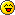wavey
HeavyweightPosts : 108
Join date : 2012-01-17
Age : 23
Location : ...secretSuvat Expressions

Suvat ... what is it? =o
Suvat is actually letters that mechanics use.. abbreviations if you like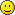S = distance
U = initial velocity
V = final velocity
A = acceleration, also G is the same thing = Gravitational pull
T = time

The expression you use is:
V = u + at
S = u+v /2 x t

S = ut + 1/2at(2)
v(2) = u(2) + 2aswavey
HeavyweightPosts : 108
Join date : 2012-01-17
Age : 23
Location : ...secretRe: Mathmatics revision guide Gcses to additional maths^^ i am yr 11 ad maths grp plz ask me questions take it in mind im nt a genius tho =o

Used this to help do my maths hmwk tyBeflop
Team Player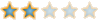Posts : 54
Join date : 2011-11-28
Age : 22
Location : Staffordshireaww thats cool~

Beflop wrote:Used this to help do my maths hmwk tyxD glad it helped.wavey
HeavyweightPosts : 108
Join date : 2012-01-17
Age : 23
Location : ...secretwhat does suvat questions look like? ;)

1) in each following cases
(a) find expressions for the velocity and acceleration at time t
(i) s = 5t(2) - t - 3
to find V and A, we differentiateplease scroll up to find what differentiation is.
to find V:
5t(2) - t - 3
10t - 1
to find A:
10t - 1
10.wavey
HeavyweightPosts : 108
Join date : 2012-01-17
Age : 23
Location : ...secretRe: Mathmatics revision guide Gcses to additional maths^^ i am yr 11 ad maths grp plz ask me questions take it in mind im nt a genius tho =o

cool...Do we have budding mathemtitions here..Wolly
HeavyweightPosts : 119
Join date : 2011-11-25
Location : UKlol this is 2nd page~?

ahh.. it spilled over to 2nd page... hope this is helping though.wavey
HeavyweightPosts : 108
Join date : 2012-01-17
Age : 23
Location : ...secretRe: Mathmatics revision guide Gcses to additional maths^^ i am yr 11 ad maths grp plz ask me questions take it in mind im nt a genius tho =o